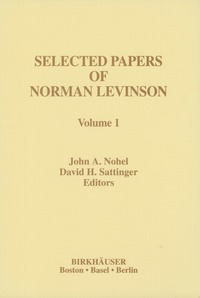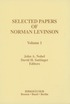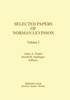Fler böcker inom
Format
Inbunden (Hardback)
Språk
Engelska
Antal sidor
1085
Utgivningsdatum
1997-12-01
Upplaga
1996 ed.
Förlag
Birkhauser Boston Inc
Medarbetare
Nohel, John A. (red.)/Sattinger, David H. (red.)/Rota, Gian-Carlo (red.)
Illustrationer
LXIII, 1085 p. In 2 volumes, not available separately.
Volymtitel
v. 1
Dimensioner
254 x 178 x 32 mm
Vikt
1217 g
Antal komponenter
2
Komponenter
2 Hardbacks
ISBN
9780817638627

### Du kanske gillar# Selected Papers of Norman Levinson

Inbunden Engelska, 1997-12-01
2819
Skickas inom 10-15 vardagar.
Fri frakt inom Sverige för privatpersoner.
Finns även som
• Skickas inom 10-15 vardagar
3409
• Skickas inom 10-15 vardagar
1549
• Skickas inom 10-15 vardagar
1979
The deep and original ideas of Norman Levinson have had a lasting impact on fields as diverse as differential & integral equations, harmonic, complex & stochas tic analysis, and analytic number theory during more than half a century. Yet, the extent of his contributions has not always been fully recognized in the mathematics community. For example, the horseshoe mapping constructed by Stephen Smale in 1960 played a central role in the development of the modern theory of dynami cal systems and chaos. The horseshoe map was directly stimulated by Levinson's research on forced periodic oscillations of the Van der Pol oscillator, and specifi cally by his seminal work initiated by Cartwright and Littlewood. In other topics, Levinson provided the foundation for a rigorous theory of singularly perturbed dif ferential equations. He also made fundamental contributions to inverse scattering theory by showing the connection between scattering data and spectral data, thus relating the famous Gel'fand-Levitan method to the inverse scattering problem for the Schrodinger equation. He was the first to analyze and make explicit use of wave functions, now widely known as the Jost functions. Near the end of his life, Levinson returned to research in analytic number theory and made profound progress on the resolution of the Riemann Hypothesis. Levinson's papers are typically tightly crafted and masterpieces of brevity and clarity. It is our hope that the publication of these selected papers will bring his mathematical ideas to the attention of the larger mathematical community.

## Passar bra ihop

1.2. +
3.De som köpt den här boken har ofta också köpt Selected Works of Norman Levinson av John Nohel, David Sattinger, G-C Rota (inbunden).

## Kundrecensioner

Har du läst boken? Sätt ditt betyg »

## Innehållsförteckning

- Volume 1.- I. Stability and Asymptotic Behavior of Solutions of Ordinary Differential Equations.- Commentary on [L 31] and [L 36].- [L 20] The Growth of the Solutions of a Differential Equation (1941).- [L 24] The Growth of the Solutions of a Differential Equation (1942).- [L 31] The Asymptotic Behavior of a System of Linear Differential Equations (1946).- [L 36] The Asymptotic Nature of Solutions of Linear Systems of Differential Equations (1948).- [L 40] On Stability of Non-Linear Systems of Differential Equations (1949).- [L 68] On u? + (1 + ? g(x)) u = 0 for ?0? | g(x)|dx < ? (1959).- II. Nonlinear Oscillations and Dynamical Systems.- Commentary on [L 29], [L 38] and [L 47].- [L 23] A General Equation for Relaxation Oscillations (1942).- [L 25] On the Existence of Periodic Solutions for Second Order Differential Equations with a Forcing Term (1943).- [L 29] Transformation Theory of Non-Linear Differential Equations of the Second Order (1944) and Correction (1948).- [L 38] A Second Order Differential Equation with Singular Solutions (1949).- [L 47] Small Periodic Perturbations of an Autonomous System with a Stable Orbit (1950).- [L 52] Forced Periodic Solutions of a Stable Non-Linear System of Differential Equations (1951).- [L 57] On the Non-Uniqueness of Periodic Solutions for an Asymmetric Lienard Equation (1952).- III. Inverse Problems for Sturm-Liouville and Schroedinger Operators.- Commentary on [L 41], [L 43] and [L 58].- [L 41] On the Uniqueness of the Potential in a Schroedinger Equation for a Given Asymptotic Phase (1949).- [L 42] Determination of the Potential from the Asymptotic Phase (1949).- [L 43] The Inverse Sturm-Liouville Problem (1949).- [L 58] Certain Explicit Relationships between Phase Shift and Scattering Potential (1953).- IV. Eigenfunction Expansions and Spectral Theory for Ordinary Differential Equations.- Commentary on [L 49], [L 51], and [L 59].- [L 39] Criteria for the Limit-Point Case for Second Order Linear Differential Operators (1949).- [L 49] A Simplified Proof of the Expansions Theorem for Singular Second Order Linear Differential Equations (1951).- [L 50] Addendum to "A Simplified Proof of the Expansions Theorem for Singular Second Order Linear Differential Equations" (1951).- [L 51] On the Nature of the Spectrum of Singular Second Order Linear Differential Equations (1951).- [L 53] The L-Closure of Eigenfunctions Associated with Selfadjoint Boundary Value Problems (1952).- [L 59] The Expansion Theorem for Singular Self-Adjoint Linear Differential Operators (1954).- [L 65] Transform and Inverse Transform Expansions for Singular Self-Adjoint Differential Operators (1958).- V. Singular Perturbations of Ordinary and Partial Differential Equations.- Commentary on [L 45], [L 48], [L 60], [L 62], [L 63], [L 67], [L 56] and [L 46].- [L 45] Perturbations of Discontinuous Solutions of Non-Linear Systems of Differential Equations (1950).- [L 48] An Ordinary Differential Equation with an Interval of Stability, a Separation Point, and an Interval of Instability (1950).- [L 60] Singular Perturbations of Non-Linear Systems of Differential Equations and an Associated Boundary Layer Equation (1954).- [L 62] Periodic Solutions of Singularly Perturbed Systems (1955).- [L 56] A Boundary Value Problem for a Nonlinear Differential Equation with a Small Parameter (1952).- [L 63] A Boundary Value Problem for a Singularly Perturbed Differential Equation (1955).- [L 67] A Boundary Value Problem for a Singularly Perturbed Differential Equation (1958).- [L 46] The First Boundary Value Problem for ??u +A(x,y)ux + B(x,y)uy + C(x, y)u = D(x,y) for small ? (1950).- VI. Elliptic Partial Differential Equations.- Commentary on [L 75], [L 78], [L87].- [L 75] Positive Eigenfunctions for ?u + ?f(u) = 0 (1962).- [L 78] Dirichlet Problem for ?u = f(P, u) (1963).- [L 87] One-Sided Inequalities for Elliptic Differential Operators (1965).- VII. Integral Equations.- Commentary on [L 73].- [L 32] On the Asymptotic Sh